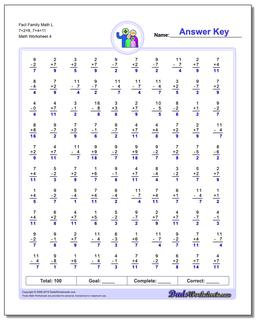# Math Worksheets: Fact Family Math: Fact Family Math: Fact Family Math L 7+2=9, 7+4=11 (Fourth Worksheet)## Fact Family Math L 7+2=9, 7+4=11 (Fourth Worksheet)

PropertyValue
DescriptionFact Family Math L 7+2=9, 7+4=11: These math worksheets have 100 addition and subtraction fact family problems and make for a challenging two minute test. (Fourth Worksheet)
Resource TypeWorksheet